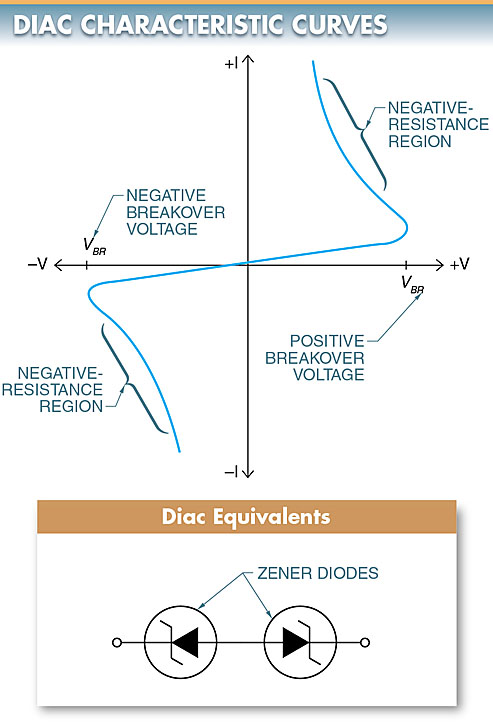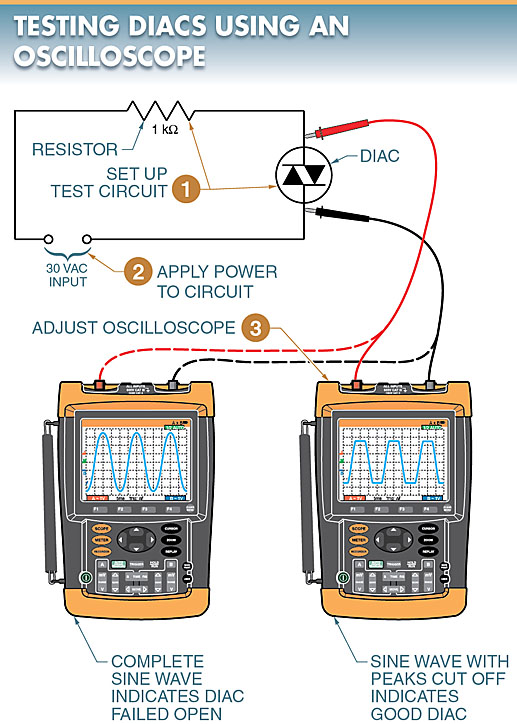# Diac: Operation, Applications, Testing

Want create site? Find Free WordPress Themes and plugins.

A diac is a three-layer, two-terminal bidirectional device that is typically used as a triggering device to control the gate current of a triac. See Figure 1. A diac is a special diode that can be triggered into conduction in either direction.Figure 1 A diac is a three-layer, two-terminal bidirectional device.

## Diac Operation

Electrically, a diac operates in a manner similar to two zener diodes that are connected in series in opposite directions.

The diac is used primarily as a triggering device. This operation is accomplished by the use of the negative resistance characteristic of the diac (current decreases with an increase of applied voltage).

A diac has negative resistance because it does not conduct current until the voltage across it reaches breakover voltage.

When a positive or negative voltage reaches the breakover voltage, the diac rapidly switches from a high-resistance state to a low-resistance state. See Figure 2.

Since the diac is a bidirectional device, it is ideal for controlling a triac, which is also bidirectional.

## Diac Applications

The gate-control circuits of triacs can be improved by adding a breakover device in the gate lead, such as a diac.

Using a diac in the gate-triggering circuit offers an important advantage over simple gate-control circuits. The advantage is that the diac delivers a pulse of gate current rather than a sinusoidal gate current. This results in a better-controlled firing sequence. Thus, diacs are used almost exclusively as triggering devices.Figure 2. Diac Characteristic Curve and Equivalent Circuit

## Universal Motor Speed Controllers

A diac/triac combination can be used to control the power to a universal motor. See Figure 3.

In the circuit, capacitor C1 charges up to the firing voltage of the diac in either direction. Once fired, the diac applies a voltage to the gate of the triac. The triac conducts and supplies power to the motor.Figure 3. speed control of universal motor using diac and triac

Note: The triac will conduct in either direction. Since the universal motor is basically a series DC motor, the current flowing in either direction will cause rotation in only one direction. The speed may be changed by varying the resistance of potentiometer R1, which in turn varies the RC time constant.

## Testing Diacs using DMM & Oscilloscope

A digital multimeter (DMM) may be used to test a diac for a short circuit. See Figure 4. To test a diac for a short circuit, the following procedure is applied:

1. Set the DMM on the Ω scale.Figure 4. A DMM may be used to test a diac for a short circuit.

Both resistance readings should show high resistance because the diac is essentially two zener diodes connected in series. Testing a diac in this manner only shows that the component is shorted. If a diac is suspected of being open, it should be tested using an oscilloscope. See Figure 5. To test a diac using an oscilloscope, the following procedure is applied:

1. Set up the test circuit.
2. Apply power to the circuit.